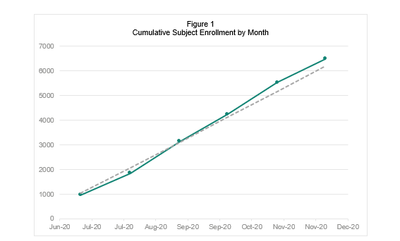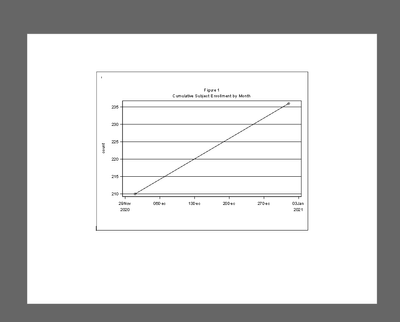## how to add line colors in proc sgplot

HI,

Several things i want to do:

1. how to remove yaxis label ("count") without removing yaxis grid?  I tried YAXIS DISPLAY=(NOLABEL) but it removed both label and grid lines

2.  How to add colors on line graph on .rtf file (example shown below)

3. How to have y-axis start at "0" instead of "210"?

4. how to expand graph bigger so that it can fit the page better

thank you!

want:Current code:

``````
Proc sgplot data=work.toplot noautolegend;
where enrlfl='Y';
reg x=enrldt y=count / lineattrs=(pattern=shortdash);
series x=enrldt  y=count;
yaxis grid;
XAXIS DISPLAY=(NOLABEL);
run;   ``````

current output:4 REPLIES 4

## Re: how to add line colors in proc sgplot

@HitmonTran wrote:

HI,

Several things i want to do:

1. how to remove yaxis label ("count") without removing yaxis grid?  I tried YAXIS DISPLAY=(NOLABEL) but it removed both label and grid lines

2.  How to add colors on line graph on .rtf file (example shown below)

3. How to have y-axis start at "0" instead of "210"?

4. how to expand graph bigger so that it can fit the page better

thank you!

want:Current code:

``````
Proc sgplot data=work.toplot noautolegend;
where enrlfl='Y';
reg x=enrldt y=count / lineattrs=(pattern=shortdash);
series x=enrldt  y=count;
yaxis grid;
XAXIS DISPLAY=(NOLABEL);
run;   ``````

current output:1. how to remove yaxis label ("count") without removing yaxis grid?  I tried YAXIS DISPLAY=(NOLABEL) but it removed both label and grid lines:       Add Label = " " to the yaxis.

2.  How to add colors on line graph on .rtf file (example shown below): Color which lines? What color?

you can specify a color for the graphed line by adding to the lineattrs=(pattern=shortdash color=Blue)

The default colors are set based on the currently active ODS Style

3. How to have y-axis start at "0" instead of "210"? Specify values.      Add to the Yaxis statement. something like Values=(0 to 250 by 50) which places a tick mark at 0, 50, 100, ... 250 ( I prefer positive control when possible) or the option MIN=0

4. how to expand graph bigger so that it can fit the page better   The size of the graph is set using the ODS Graphics options. Such as ODS Graphics / height=5in width=5in ; would set the display area to 5x5 inches. You can use other units of measure like CM, MM, pct (percentage). Note that changing both height and width can result in an aspect change and distort fonts and by default larger area will use larger font sizes. There are about 20 options. I'm not going to go through the list. Look up "ods graphics" statement in the documentation.

## Re: how to add line colors in proc sgplot

sorry i am fairly new to SAS,

when i add label='  '  on yaxis statement, it removes the grid lines.

``````Proc sgplot data=work.toplot noautolegend;
where enrlfl='Y';
reg x=enrldt y=count / lineattrs=(pattern=shortdash);
series x=enrldt y=count / lineattrs=(color=teal);
yaxis grid label='';
XAXIS DISPLAY=(NOLABEL);
run;``` ```

## Re: how to add line colors in proc sgplot

@HitmonTran wrote:

sorry i am fairly new to SAS,

when i add label='  '  on yaxis statement, it removes the grid lines.

``````Proc sgplot data=work.toplot noautolegend;
where enrlfl='Y';
reg x=enrldt y=count / lineattrs=(pattern=shortdash);
series x=enrldt y=count / lineattrs=(color=teal);
yaxis grid label='';
XAXIS DISPLAY=(NOLABEL);
run;``` ```

May be a version difference. On my system the grid lines stay.

## Re: how to add line colors in proc sgplot

It'll be easier for us to communicate if we use the same data. Here is some code that creates a graph for some data that are distributed with SAS. Paste the graph that results.

``````Proc sgplot data=sashelp.air noautolegend;
reg x=Date y=Air / lineattrs=(pattern=shortdash);
series x=Date y=Air / lineattrs=(color=teal);
yaxis grid display=(nolabel);
xaxis display=(nolabel);
run; ``````

To address the "what version do have" question, run this command
%put &=SYSVLONG4;

and paste the results from the log. It will look something like this:

SYSVLONG4=9.04.01M6P11072018

Discussion stats
• 4 replies
• 724 views
• 2 likes
• 3 in conversation